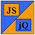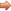# JavaScript & jQuery Tutorials

JS HomeJS ReferenceMember

## Member operators

Access members of an object.

### Description

Member operators allow access to the properties and methods of an object.

• Under the hood properties and methods are the same thing. A method is a property with a function for it's value.
• We can use dot or bracket notation to access the members of an object.

### Dot Notation

The first way of accessing the members of an object is through dot notation.

### Syntax

Signature Description
`anObject.property`Get an object property.
`anObject.property = setProperty`Set an object property.

### Parameters

Parameter Description
`property`A valid JavaScript identifier.
• Only letters, numbers, the dollar (\$) and underscore (_) symbols are allowed.
• Only letters, the dollar (\$) and underscore (_) symbols are allowed as the first character.

### Examples

Lets see some examples of using dot notation.

``````

// Store properties in an array.
var dotProps = new Array(6);

dotProps = Object.prototype + '\n';
dotProps = Object.constructor + '\n';
dotProps = Math.E + '\n';
dotProps = Math.PI + '\n';
dotProps = Object.toString() + '\n';
dotProps = Date.parse();

``````

Press the button below to action the above code:

### Bracket Notation

The second way of accessing the members of an object is through bracket notation.

### Syntax

Signature Description
`anObject[property]`Get an object property.
`anObject[property] = setProperty`Set an object property.

### Parameters

Parameter Description
`property`A string that isn't constrained by the naming limitations of dot notation.

### Examples

Lets see some examples of using bracket notation.

``````

// Store properties in an array.
var bracketProps = new Array(6);

bracketProps = Object['prototype'] + '\n';
bracketProps = Object['constructor'] + '\n';
bracketProps = Math['E'] + '\n';
bracketProps = Math['PI'] + '\n';
bracketProps = Object['toString'] + '\n';
bracketProps = Date['parse'];

``````

Press the button below to action the above code:

## Related Tutorials

JavaScript Basic Tutorials - Lesson 7 - Objects